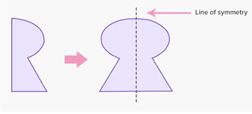# Line Symmetry Worksheets

What is Line Symmetry? We often find symmetrical things around us. We call an object symmetrical to the other one when there is an exact reflection or mirror image. A particular object, line, or shape is formed. The line of symmetry can be defined as the axis or an imaginary line that passes through an object or shape dividing it into two identical and equal parts. For instance, we have the following shapes:Here, the dotted line is the line of symmetry. The above shapes are divided by the line of symmetry and these figures have an exact resemblance to each other. They are also divided into two equal parts.

• ### Basic Lesson

Guides students through identifying line symmetry with real world applications. Line symmetry is two halves of figure mirror each other across a line. The line of symmetry is line that divides the figure into two mirror images. A simple test to determine line symmetry of a figure is to fold the figure along the supposed line of symmetry and check the two halves of the figure coincide.

• ### Intermediate Lesson

Demonstrates how to identify horizontal and vertical symmetry. Certain geometric figures demonstrate the concept of reflections and line symmetry. This figure has two lines of symmetry, shown by the red lines.

• ### Independent Practice 1

A really great activity for allowing students to understand the concepts of the Line Symmetry. Classify horizontal and vertical line symmetry.

• ### Independent Practice 2

Students determine the Line Symmetry in 20 assorted problems. The answers can be found below.

• ### Homework Worksheet

Students are provided with 12 problems to achieve the concepts of Line Symmetry.

• ### Skill Quiz

This tests the students ability to understand Line Symmetry.

• ### Homework and Quiz Answer Key

Answers for the homework and quiz.

• ### Lesson and Practice Answer Key

Answers for both lessons and both practice sheets.

• ### Basic Lesson

Guides students through solving transformations via line symmetry exercises. The piece of artwork displays line symmetry in picture. The line of symmetry divides the picture into mirror images.

• ### Intermediate Lesson

Demonstrates the concept of advanced skill while solving transformations via line symmetry exercises. Certain geometric figures demonstrate the concept of reflections and line symmetry. This figure has two lines of symmetry, shown by the red lines.

• ### Independent Practice 1

A really great activity for allowing students to understand the concepts of the transformations via line symmetry exercises.

• ### Independent Practice 2

Students use transformations via line symmetry exercises in 20 assorted problems. The answers can be found below.

• ### Homework Worksheet

Students are provided with 12 problems to achieve the concepts of transformations via line symmetry.

• ### Skill Quiz

This tests the students ability to understand transformations via line symmetry exercises.